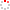CIOMP OpenIR  > 中科院长春光机所知识产出
 Modeling and Analysis of a Multi-Degree-of-Freedom Micro-Vibration Simulator Wang, X. M.; Z. B. Xu; S. He; Q. W. Wu; H. Li; Y. P. Zhao and L. He 2017 Source Publication Shock and VibrationAbstract To reproduce the disturbance forces and moments generated by the reaction/momentum wheel assembly, a multi-degree-offreedom micro-vibration simulator is proposed. This can be used in the ground vibration experiments of an optical payload replacing the real action/momentum wheel assembly. First, the detailed structure of the micro-vibration simulator is introduced. Then, the complete system kinematic and dynamicmodels of the micro-vibration simulator are derived. In addition, the disturbance forces andmoments produced by the micro-vibration simulator are calculated. Finally, the normalmode analysis and a cosimulation are adopted to verify the validity of this method. The analysis and simulation results show that the micro-vibration simulator can exactly reproduce the disturbance forces and moments with different amplitudes and different frequency ranges. Indexed By sci ; ei Language 英语 Document Type 期刊论文 Identifier http://ir.ciomp.ac.cn/handle/181722/59292 Collection 中科院长春光机所知识产出 Recommended CitationGB/T 7714 Wang, X. M.,Z. B. Xu,S. He,et al. Modeling and Analysis of a Multi-Degree-of-Freedom Micro-Vibration Simulator[J]. Shock and Vibration,2017. APA Wang, X. M.,Z. B. Xu,S. He,Q. W. Wu,H. Li,&Y. P. Zhao and L. He.(2017).Modeling and Analysis of a Multi-Degree-of-Freedom Micro-Vibration Simulator.Shock and Vibration. MLA Wang, X. M.,et al."Modeling and Analysis of a Multi-Degree-of-Freedom Micro-Vibration Simulator".Shock and Vibration (2017).